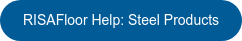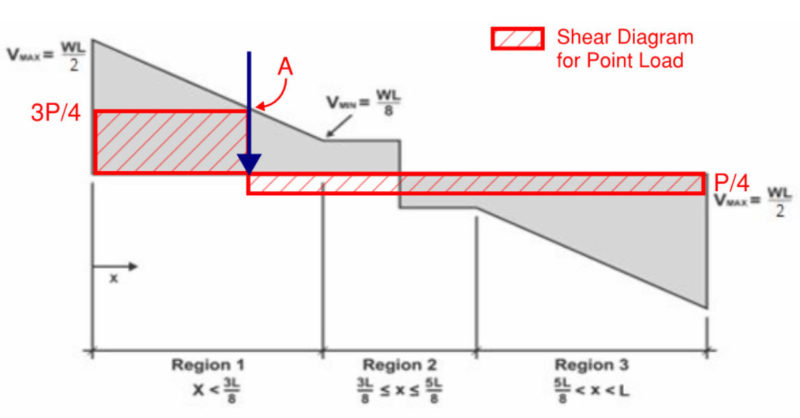# Equivalent Uniform Load Method in Steel Joist Design under…

RISAFloor will choose a joist such that the capacity shear envelope of the joist under a standard uniform load is still greater than the actual shear at all locations along the length of the joist. Because the joist will have a shear strength greater than zero at the midspan of the beam, the shear envelope for a joist with a maximum uniform load capacity of W, would be given by the figure shown below: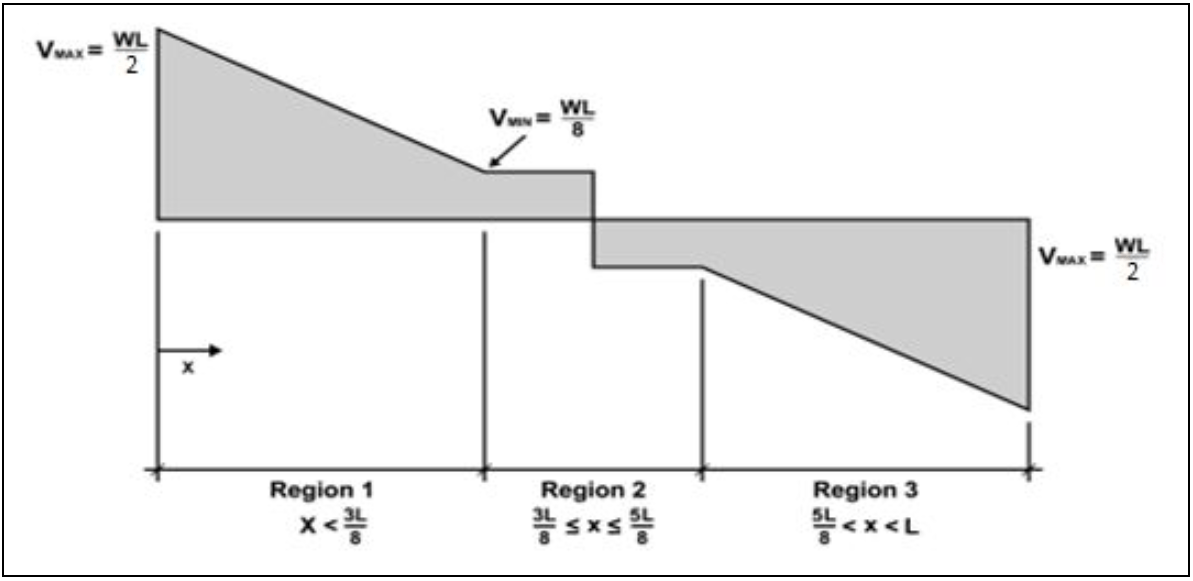In addition to checking the shear envelope, the program will make sure that the maximum allowable moment is not exceeded along the length of the joist. The maximum moment is defined as WL2/8, where W is the maximum allowable uniform load for that joist and span that is listed in the joist tables.

Below is an example of a 22K4 joist that is subject to both Uniform Dead Load (UDL), Uniform Live Load (ULL), as well as a 1 kip point Dead Load applied at quarter-span.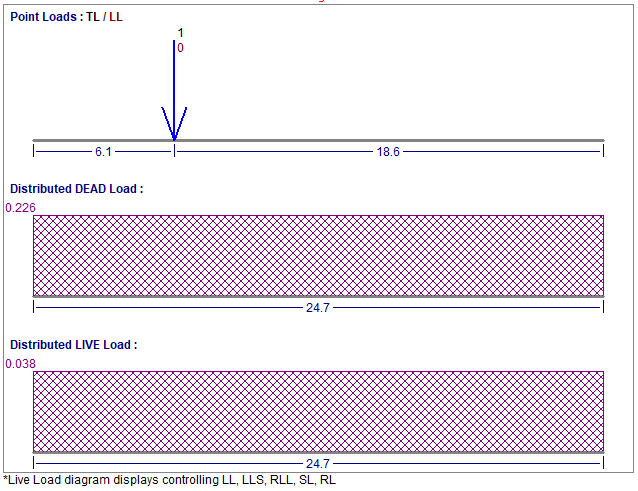When determining the force demand of the steel joist, the program calculates the equivalent uniform load for the point load, then adds it to the existing UDL or ULL to obtain the total uniform load. Note in this case the point load is Dead Load, so the equivalent uniform load will be added to UDL.

To calculate the equivalent uniform load, the shear diagram of the point load shall not exceed the shear envelope. The governing point A is at Region 1 as shown below: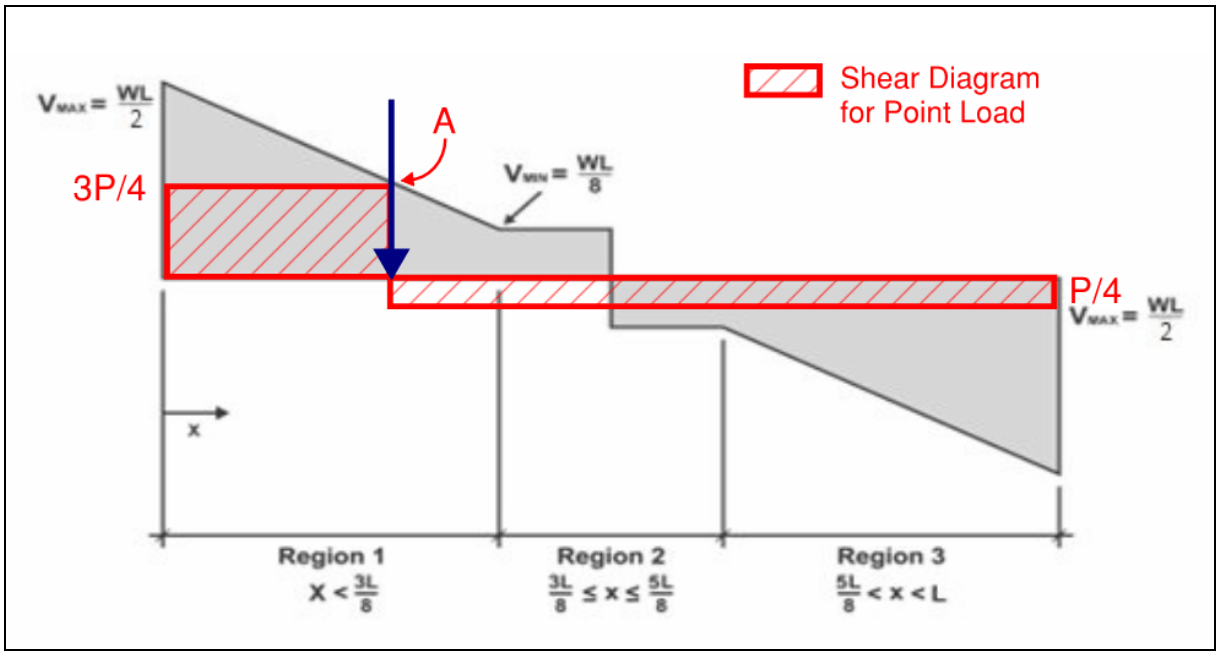The point A has the shear force of 3*P/4 per the shear diagram. Using the geometry and shear envelope values, the shear capacity at point A can be calculated as WL/4. This corresponds to the shear envelope of the smallest joist that satisfies the shear demand of the point load. Therefore the equivalent uniform load for the point load can be back-calculated as W = (3*P/4)*(4/L) = 3*P/L = 3*(1 kip)/(24.7 ft) = 0.121 klf.

Similarly, the maximum moment demand from the point load 3*P*L/16 is checked against the maximum moment capacity WL2/8. And the equivalent uniform load due to moment W = 1.5*P/L=0.061 klf, which is smaller than the value from shear check, therefore the governing equivalent uniform load for this point load is 0.121 klf.

So the total UDL = 0.226 klf + 0.121 klf = 0.347 klf which is very close to the design value reported by RISAFloor in the design of the steel joist.For more information about steel products (steel joists, joist girders and composite steel joists) in RISAFloor, visit the RISAFloor Help File: